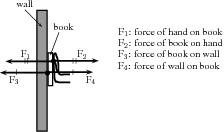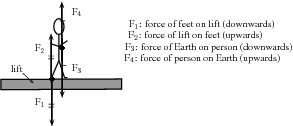# 11.4 Newton's third law

 Page 1 / 1

## Newton's third law of motion

Newton's Third Law of Motion deals with the interaction between pairs of objects. For example, if you hold a book up against a wall you are exerting a force on the book (to keep it there) and the book is exerting a force back at you (to keep you from falling through the book). This may sound strange, but if the book was not pushing back at you, your hand would push through the book! These two forces (the force of the hand on the book (F ${}_{1}$ ) and the force of the book on the hand (F ${}_{2}$ )) are called an action-reaction pair of forces. They have the same magnitude, but act in opposite directions and act on different objects (the one force is onto the book and the other is onto your hand).

There is another action-reaction pair of forces present in this situation. The book is pushing against the wall (action force) and the wall is pushing back at the book (reaction). The force of the book on the wall (F ${}_{3}$ ) and the force of the wall on the book (F ${}_{4}$ ) are shown in the diagram.Newton's action-reaction pairs
Newton's Third Law of Motion

If body A exerts a force on body B, then body B exerts a force of equal magnitude on body A, but in the opposite direction.

Newton's action-reaction pairs can be found everywhere in life where two objects interact with one another. The following worked examples will illustrate this:

Dineo is seated in the passenger seat of a car with the seat belt on. The car suddenly stops and he moves forwards until the seat belt stops him. Draw a labeled force diagram identifying two action-reaction pairs in this situation.

1. Start by drawing the picture. You will be using arrows to indicate the forces so make your picture large enough so that detailed labels can also be added. The picture needs to be accurate, but not artistic! Use stickmen if you have to.

2. Take one pair at a time and label them carefully. If there is not enough space on the drawing, then use a key on the side.

Tammy travels from the ground floor to the fifth floor of a hotel in a lift. Which ONE of the following statements is TRUE about the force exerted by the floor of the lift on Tammy's feet?

1. It is greater than the magnitude of Tammy's weight.
2. It is equal in magnitude to the force Tammy's feet exert on the floor.
3. It is equal to what it would be in a stationary lift.
4. It is greater than what it would be in a stationary lift.
1. This is a Newton's Third Law question and not Newton II. We need to focus on the action-reaction pairs of forces and not the motion of the lift. The following diagram will show the action-reaction pairs that are present when a person is standing on a scale in a lift.Newton's action-reaction pairs in a lift

In this question statements are made about the force of the floor (lift) on Tammy's feet. This force corresponds to F ${}_{2}$ in our diagram. The reaction force that pairs up with this one is F ${}_{1}$ , which is the force that Tammy's feet exerts on the floor of the lift. The magnitude of these two forces are the same, but they act in opposite directions.

2. It is important to analyse the question first, before looking at the answers as the answers might confuse you. Make sure that you understand the situation and know what is asked before you look at the options.

The correct answer is B.

Tumi presses a book against a vertical wall as shown in the sketch.

1. Draw a labelled force diagram indicating all the forces acting on the book.
2. State, in words, Newton's Third Law of Motion.
3. Name the action-reaction pairs of forces acting in the horizontal plane.
1. A force diagram will look like this:

Note that we had to draw all the forces acting on the book and not the action-reaction pairs. None of the forces drawn are action-reaction pairs, because they all act on the same object (the book). When you label forces, be as specific as possible, including the direction of the force and both objects involved, for example, do not say gravity (which is an incomplete answer) but rather say 'Downward (direction) gravitational force of the Earth (object) on the book (object)'.

2. If body A exerts a force onto body B, then body B will exert a force equal in magnitude, but opposite in direction, onto body A.

3. The question only asks for action-reaction forces in the horizontal plane. Therefore:

Pair 1: Action: Applied force of the girl on the book; Reaction: The force of the book on the girl.

Pair 2: Action: Force of the book on the wall; Reaction: Force of the wall on the book.

Note that a Newton III pair will always involve the same combination of words, like 'book on wall' and 'wall on book'. The objects are 'swapped around' in naming the pairs.

## Experiment : balloon rocket

Aim:

In this experiment for the entire class, you will use a balloon rocket to investigate Newton's Third Law. A fishing line will be used as a track and a plastic straw taped to the balloon will help attach the balloon to the track.

Apparatus:

You will need the following items for this experiment:

1. balloons (one for each team)
2. plastic straws (one for each team)
3. tape (cellophane or masking)
4. fishing line, 10 meters in length
5. a stopwatch - optional (a cell phone can also be used)
6. a measuring tape - optional

Method:

1. Divide into groups of at least five.
2. Attach one end of the fishing line to the blackboard with tape. Have one teammate hold the other end of the fishing line so that it is taut and roughly horizontal. The line must be held steady and must not be moved up or down during the experiment.
3. Have one teammate blow up a balloon and hold it shut with his or her fingers. Have another teammate tape the straw along the side of the balloon. Thread the fishing line through the straw and hold the balloon at the far end of the line.
4. Let go of the rocket and observe how the rocket moves forward.
5. Optionally, the rockets of each group can be timed to determine a winner of the fastest rocket.
1. Assign one teammate to time the event. The balloon should be let go when the time keeper yells “Go!” Observe how your rocket moves toward the blackboard.
2. Have another teammate stand right next to the blackboard and yell “Stop!” when the rocket hits its target. If the balloon does not make it all the way to the blackboard, “Stop!” should be called when the balloon stops moving. The timekeeper should record the flight time.
3. Measure the exact distance the rocket traveled. Calculate the average speed at which the balloon traveled. To do this, divide the distance traveled by the time the balloon was “in flight.” Fill in your results for Trial 1 in the Table below.
4. Each team should conduct two more races and complete the sections in the Table for Trials 2 and 3. Then calculate the average speed for the three trials to determine your team's race entry time.
Results:
 Distance (m) Time (s) Speed (m $·$ s ${}^{-1}$ ) Trial 1 Trial 2 Trial 3 Average:
Conclusions: The winner of this race is the team with the fastest average balloon speed.

While doing the experiment, you should think about,

1. What made your rocket move?
2. How is Newton's Third Law of Motion demonstrated by this activity?
3. Draw pictures using labeled arrows to show the forces acting on the inside of the balloon before it was released and after it was released.

## Exercise

1. A fly hits the front windscreen of a moving car. Compared to the magnitude of the force the fly exerts on the windscreen, the magnitude of the force the windscreen exerts on the fly during the collision, is ...
1. zero.
2. smaller, but not zero.
3. bigger.
4. the same.
2. Which of the following pairs of forces correctly illustrates Newton's Third Law?

#### Questions & Answers

how to calculate the relative formula for mass(m)?
Sphesihle Reply
molecule lewis structure
diesabel Reply
Can you please explain the right hand rule for solenoid
Azafunde Reply
how do I find the percentage composition of an element
Nyiko Reply
total Mr of the element ÷total Mr of the compound all ×100
Ndimbonile
no
Globel
how to calculate distance between two charges
Kefentse Reply
how
Globel
what is a limiting reagent?
Sizwe Reply
it is a reactant that gets used up completely after a reaction
Bongy
Two charges of + 3nC and -5 nC are separated by a distance of 40cm. what is the electrostatic force between the two charges
Lethabo Reply
3,37×10^-6
Refilwe
how do you determine electron configuration?
Karabo Reply
A chemist at a water purification plant adds 700g of Al2(SO4)3 to a sample of water calculate the maximum mass of Al(OH)3 that can be produced from this mass of Al2(SO4)3
Akhonke Reply
how to calculate the mass of a sphere in ekectrostatics
Mpilo Reply
although the shape of molecules of H2S and H2O is similar,there is a remarkable difference in their boiling point explain the statement
Kgopotso Reply
What is coulomb's law
Boitumelo Reply
?
Rethabile
The amount of force between two stationary, electrically charged particles.
Olerato
Coulombs law is the magnitude of electrostatic force that one charge exerts on the other and is directly proportional to the product of the charges and inversely proportional to the squared distance between them
Refilwe
how to identify the type of intermolecular forces in pairs of molecules
Okheka Reply
How many gramd of NO2 are produced from 30g of NO
DEVAN Reply
what is orbitals?
Juvilyn Reply
a specification of the energy and probability density of an electron at any point in an atom or molecule
Dineo

### Read also:

#### Get Jobilize Job Search Mobile App in your pocket Now!

Source:  OpenStax, Siyavula textbooks: grade 11 physical science. OpenStax CNX. Jul 29, 2011 Download for free at http://cnx.org/content/col11241/1.2
Google Play and the Google Play logo are trademarks of Google Inc.

Notification Switch

Would you like to follow the 'Siyavula textbooks: grade 11 physical science' conversation and receive update notifications?By Stephen VoronBy Sarah WarrenBy Jonathan LongBy Sarah WarrenBy Ellie BanfieldBy Joli JuliannaBy OpenStaxByBy OpenStaxBy OpenStax